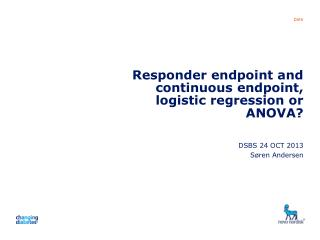DownloadDownload PresentationResponder endpoint and continuous endpoint, logistic regression or ANOVA?

# Responder endpoint and continuous endpoint, logistic regression or ANOVA?

Télécharger la présentation## Responder endpoint and continuous endpoint, logistic regression or ANOVA?

- - - - - - - - - - - - - - - - - - - - - - - - - - - E N D - - - - - - - - - - - - - - - - - - - - - - - - - - -
##### Presentation Transcript

1. Presentation title Responder endpoint and continuous endpoint, logistic regression or ANOVA? DSBS 24 OCT 2013 Søren Andersen

2. Presentation title Example and problem • HbA1c is analysed with an ANCOVA model and in addition the ”responder rate” (HbA1c < 7%) is analysed by a logistic regression model • Well documented that dichotomising reduces sensitivity • Results presented as difference in HbA1c and as odds ratio • Difficult to compare the results • Difficult to interpret odds-ratio for probalities p (from logistic regression model) in [0.2; 0.8], no interpretation as relative risk • Example: Old study with Liraglutide

3. Presentation title

4. Presentation title Outline • Comparisons on probability scale • Show no difference between logit and probit in estimated responder probabilities (and in treatment differences in responder probabilities) • Compare responder probabilites derived from ANCOVA with responder probabilities from logit and probit • Comparisons on continuous scale • Compare estimates from logit and probit to estimates from ANCOVA

5. Presentation title

6. Presentation title Suggestion: use probit instead of logit • A probit model for binary data is very similar to a logit model. Very difficult to discriminate between the two. • Pro logit: • a logit model is very useful for retrospective studies (not the case here) • a logit model is convenient for calculation of conditional probabilities • a logit model offers interpretation in terms of odds-ratio • Technical point: simple sufficient statistics • Pro probit: • offers interpretation in terms of a latent normal variable (threshold model)

7. Presentation title Comparions of logit and probit estimates of probabilities • Logit and probit model with effects of • Country (17) • Pre-treatment (2) • Treatment (3) • Base line HbA1c • responder probabilitieswereestimated for all countries (17) and pre-treatment (2), treatments (3) and 3 values of base line HbA1c (mean +- std) • In all 17 x 2 x 3 x 3 = 306 probabilities

8. Presentation title Estimated p’s of 3 treatments across subgroups

9. Presentation title Presentation of results from probit and logit models • Present differences in estimated proportions between two treatment groups, Lira and Comparator – not constant • Depend on proportion in the Lira (or Comparator)

10. Presentation title

11. Presentation title Comparing logit and probit treatment differences

12. Presentation title Estimated p’s of 3 treatments across subgroups ANCOVA and probit

13. Presentation title

14. Presentation title

15. Presentation title

16. Presentation title Comparison on “latent scale” of parameter estimates

17. Presentation title Comparison of estimates of treatment difference • From ANCOVA : 0.2367 (residual s = 0.81) • From probit: 0.3379 (”residual s = 1”) • 0.3379*0.81 = 0.2758 • From logit: 0.5440 convert to probit: 0.5440*0.607 = 0.3302 convert to ANCOVA: 0.3302*0.81 = 0.2695 To obtain the same precision of estimate from probit and logit as for ANCOVA twice as many observations areneeded

18. Presentation title Conclusions • Dichotomising reduces sensitivity (in the example sample size doubles) • Communicate results from logit/probit as difference in proportions if OR markedly different from RR • Compare results from ANCOVA and logit/probit on probability scale and on ”latent scale”

19. Presentation title Composite responder endpoint? • Responder: (HbA1c < 7) & (change in weight < 0), i.e. two binary response B1 and B2 combined • Why composite? Why collapse 3 categories of the B1 x B2 outcome? • For quantitative responses we test for each parameter: H0: no difference in HbA1c, H0: no difference in chg_bw • Analyse B1 and B2 separately or • Analyse the full response pattern B1 x B2, as marginal B1, B2 conditional on B1 (or other way round)